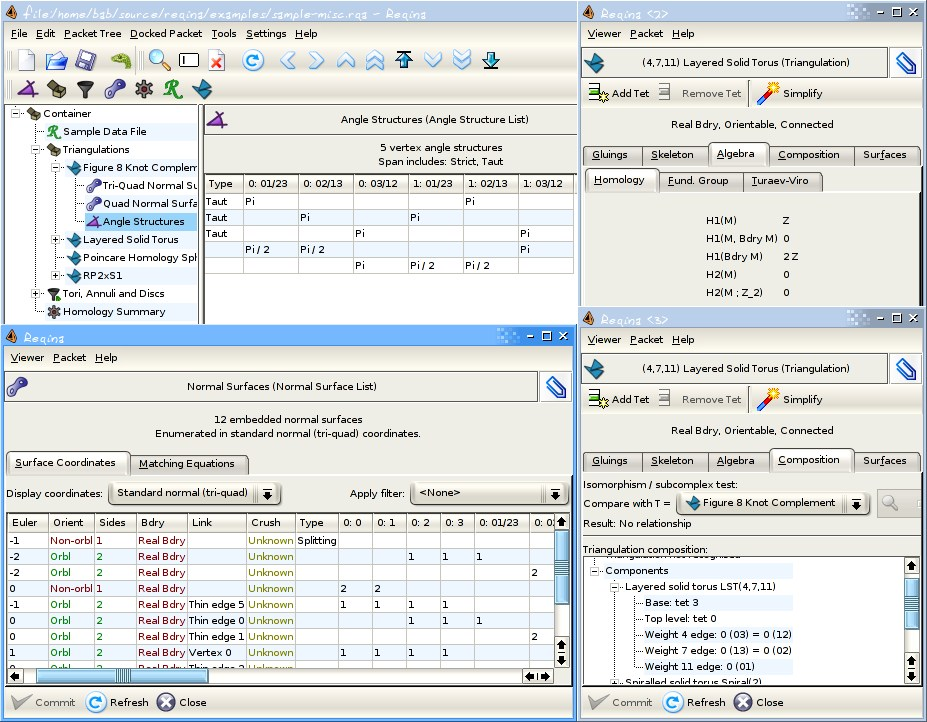## 11 Search Results

### cdd/cddplus

The program cdd+ is a C++ implementation of the Double Description Method of Motzkin et al. for generating all vertices (i.e. extreme points) and extreme rays of a general convex polyhedron in R^d given by a system of linear inequalities.

### CGAL

CGAL is a collaborative effort of several sites in Europe and Israel. The goal is to make the most important of the solutions and methods developed in computational geometry available to users in industry and academia in a C++ library. The goal is to provide easy access to useful, reliable geometric algorithms The CGAL library contains: the Kernel with geometric primitives such as points, vectors, lines, predicates for testing things such as relative positions of points, and operations such as intersections and distance calculation, the Basic Library which is a collection of standard data structures and geometric algorithms, such as convex hull in 2D/3D, (Delaunay) triangulation in 2D/3D, planar map, polyhedron, smallest enclosing circle, and multidimensional query structures, the Support Library which offers interfaces to other packages, e.g., for visualisation, and I/O, and other support facilities.

### Convex

Convex is a Maple package for convex geometry. It can deal with rational polytopes, cones and other polyhedra of arbitrary dimension. Polyhedral complexes and fans are also supported, as well as computations in face lattices. The integration into Maple makes Convex particularly suited for "applied" problems where polyhedra arise together with other mathematical structures.

### HiFlow³

HiFlow³ is a multi-purpose finite element software providing powerful tools for efficient and accurate solution of a wide range of problems modeled by partial differential equations. Based on object-oriented concepts and the full capabilities of C++ the HiFlow³ project follows a modular and generic approach for building efficient parallel numerical solvers. It provides highly capable modules dealing with the mesh setup, finite element spaces, degrees of freedom, linear algebra routines, numerical solvers, and output data for visualization. Parallelism – as the basis for high performance simulations on modern computing systems – is introduced on two levels: coarse-grained parallelism by means of distributed grids and distributed data structures, and fine-grained parallelism by means of platform-optimized linear algebra back-ends.

### Matlab

MATLAB is a high-level language and interactive environment that enables you to perform computationally intensive tasks faster than with traditional programming languages such as C, C++, and Fortran.

### Normaliz

Normaliz is a tool for computations in affine monoids, vector configurations, lattice polytopes, and rational cones. Its input data can be specified in terms of a system of generators or vertices or a system of linear homogeneous Diophantine equations, inequalities and congruences or a binomial ideal. Normaliz computes the dual cone of a rational cone (in other words, given generators, Normaliz computes the defining hyperplanes, and vice versa), a placing (or lexicographic) triangulation of a vector configuration (resulting in a triangulation of the cone generated by it), the Hilbert basis of a rational cone, the lattice points of a lattice polytope, the normalization of an affine monoid, the Hilbert (or Ehrhart) series and the Hilbert (or Ehrhart) (quasi) polynomial under a Z-grading (for example, for rational polytopes), NEW: generalized (or weighted) Ehrhart series and Lebesgue integrals of polynomials over rational polytopes via NmzIntegrate, a description of the cone and lattice under consideration by a system of inequalities, equations and congruences

### PLTMG

PLTMG is a package for solving elliptic partial differential equations in general regions of the plane. It is based on continuous piecewise linear triangular finite elements, and features adaptive local mesh refinement, multigraph iteration, and pseudo-arclength continuation options for parameter dependencies. It also provides options for solving several classes of optimal control and obstacle problems. The package includes an initial mesh generator and several graphics packages. Support for the Bank-Holst parallel adaptive meshing strategy is also provided. PLTMG is provided as Fortran (and a little C) source code, in both single and double precision versions. The code has interfaces to X-Windows, MPI, and Michael Holst's OpenGL image viewer SG. The X-Windows, MPI, and SG interfaces require libraries that are NOT provided as part of the PLTMG package.

### polymake

polymake is an object-oriented system for experimental discrete mathematics. The typical working cycle of a polymake user starts with the construction of an object of interest, auch as a convex polytope, a finite simplicial complex, a graph, etc. It is then possible to ask the system for some of the object's properties or for some form of visualization. Further steps might include more elaborate constructions based on previously defined objects. Each class of polymake objects comes with a set of rules which describe how a new property of an object can be derived from previously known ones. It is a key feature that the user can extend or modify the set of rules, add further properties or even new classes of objects (with entirely new rule bases). The functions provided include: several convex hull algorithms, face lattices of convex polytopes, Voronoi diagrams and Delaunay decompositions (in arbitrary dimensions), simplicial homology (with integer coefficients), simplicial cup and cap products, intersection forms of triangulated 4-manifolds. Several forms of (interactive) visualization via interfaces to Geomview, JavaView and other programs.

### rbMIT

The rbMIT © MIT software package implements in Matlab® all the general reduced basis algorithms. The rbMIT © MIT software package is intended to serve both (as Matlab® source) "Developers" — numerical analysts and computational tool-builders — who wish to further develop the methodology, and (as Matlab® "executables") "Users" — computational engineers and educators — who wish to rapidly apply the methodology to new applications. The rbMIT software package was awarded with the Springer Computational Science and Engineering Prize in 2009.

More information